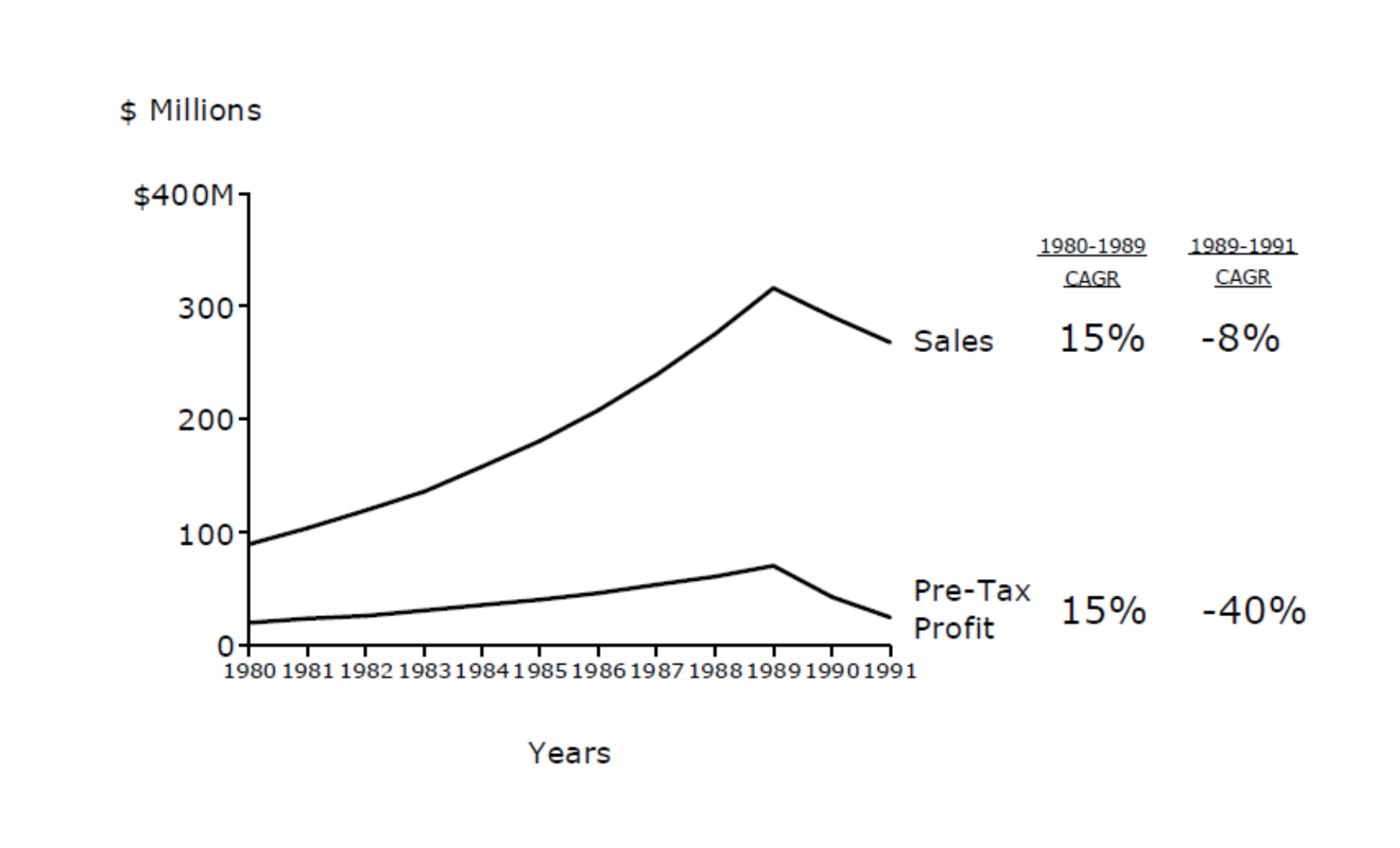# <question> If profit is decreasing at a faster rate than sales,

Case Interview case question casing consulting MBB
New answer on Aug 27, 2020
3.1 k Views

If profit is decreasing at a faster rate than are sales, why would this reflect the "fixed-cost nature of the business" (that's the answer in the case book but for some reason I thought it'd be the opposite)? Can someone help me understand what I'm supposed to draw about economies of scale from this graph and how? Thank you so much to everyone in advance!## Overview of answers

• Date ascending
• Date descending

If profit is decreasing faster than sales, it means that as the sales declines, profits decline at a greater rate. What this means is that your revenues are declining but your costs are not declining in line with the revenues to keep match.

Now as you know there are 2 types of costs - fixed and variable costs. Variable costs vary with sales - that is, as you sell more you spend more on variable costs (lets say labor or materials). However fixed costs are fixed (for at least some larger amount of time) and cannot be reduced when production reduces.

Therefore - if profits are declining at a greater rate than the sales decline it means that the costs aren't coming down proportionally and this is a fixed cost problem

The above explanation is relatively simple - it doesn't take into account many many nuances of the real world (e.g., labor is not really a fixed cost you can't hire and fire people that easily without serious repurcussions) but it would be sufficient from a logic perspective

Best,

Udayan

Hi! Thanks a lot for the explanation. I’m trying to understand this - does it make sense to think of it as: since profits are decreasing faster than are sales, the costs must be mostly fixed. Variable costs are a function of the units of sales (i.e. they change with the number of sales made), so since we’re making fewer sales, costs should also have gone down because there are fewer units sold. However, costs are increasing as we see with the decreasing profits, so they must be fixed not variable??

Almost - profits decreasing in this case does not mean costs are increasing. Fixed costs are just that - they are fixed. So costs are not decreasing as fast as sales are leading to lower than expected profits

(edited)

Hi,

Variable costs only depend on units sold. Try to image a company with only variable costs:

If you sell 10 units, let's call "r" the revenues per unit and "vc" the variable costs per unit:

Revenues = 10*r

Variable costs = 10*c

Profit = 10 * (r-c)

If you register a reduction in revenues, let's say 10% and you don't change the price per unit (meaning the revenues per unit, meaning "r"), it means that the units sold are decreased by the 10% (meaning you sell 9 units instead of 10).

You can easily see that, if the variable costs per unit remain the same, that 1. the variable costs decrease by the same amount (10%) an the profit decreases by exactly the same amount as well:

New Revenues = 9*r --> 10%

New Variable costs = 9*c --> 10%

New Profit = 9 * (r-c) --> 10%

But, if we introduce the fixed costs, let's call them "fc", as a fixed number that doesn't depend on units sold (e.g., rent, gas and electricity bills, machines, ...), you can see that if revenues decreases by 10%, costs don't decrease by the same amount, but less (because the fixed costs remain the same, no matter what), thus the profit decreases more than 10%:

New Revenues = 9*r --> 10%

New Variable costs = 9*c + fc (constant no matter what) --> <10%

New Profit = 9 * (r-c) - fc --> >10%

Please let me know if something is not clear.

Hope it helps!

To many theoretical/math-based answers here...I think this is why you're struggling!

Let's talk Lemonade :)

It costs you \$100 to operate your lemonade stand every day. (Rent the cart, rent the neighborhood curbside, pay your cashier and lemonade maker, etc.)

Every lemonade you produce costs you 50 cents in raw materials (lemons, sugar, water, cups).

If I sell my lemonade for \$1.5 a pop, that means I make \$1 per lemonade sold.

Today I sell 100 lemonades, which means I made \$100 in unit profit....but actually \$0 in pure profit (have to subtract the \$100 in fixed costs)....I earned \$0 per unit sold.

Yesterday I sold 1,000 lemonades. Which means I made \$900...and the profit per unit sold was huge...90 cents per lemonade!

Tomorrow it's raining so I'm going to sell 10 lemonades. Now, I lose \$90 and every unit sold lost me 90 cents.

See how economies of scale in a high fixed-cost environment work now? :P

(edited)